﻿ Study on the Influencing Factors of Carbon Intensity Using Skew-normal Mixed ModelPublications are Open
Access in this journal
Article Versions
Export Article
• Normal Style
• MLA Style
• APA Style
• Chicago Style
Research Article
Open Access Peer-reviewed

### Study on the Influencing Factors of Carbon Intensity Using Skew-normal Mixed Model

Ye Ren-dao , Xu Li-jun, Luo Kun, Zhang Yong
Journal of Finance and Economics. 2018, 6(1), 14-18. DOI: 10.12691/jfe-6-1-2
Published online: January 08, 2018

### Abstract

This paper firstly applies the EM algorithm and gives the maximum likelihood estimates of unknown parameters in skew-normal mixed model. For empirical analysis, we verify the skew-normal distribution characteristics of provincial carbon intensity data in China from 2000 to 2014. A skew-normal mixed model is then constructed to study the main influencing factors of carbon intensity of China. It is found that energy intensity would have the most significant influence on carbon intensity, among a group of factors including GDP per capita, proportion of secondary industry, and dependence on foreign trade. Finally, the results are compared with those based on normal mixed model, so as to confirm the statistical excellent properties of skew-normal mixed model.

### 1. Introduction

Global warming caused by excessive carbon emissions has become a serious environmental problem, and every country around the world needs to be involved in emission control. In 2015, The Chinese government submitted to the UN Framework Convention on Climate Change the document titled Enhanced Actions on Climate Change: China’s Intended Nationally Determined Contribution. According to the document, China's carbon emissions would peak around 2030, and the Chinese government would endeavor to bring down carbon intensity by 60% to 65% compared to 2005, which is no doubt a real challenge for energy conservation, emissions reduction and economic development. It is urgent to analyze the influencing factors of carbon intensity, with a view of improving emission reduction policies and achieving the development of low carbon economy in China.

Main methods applied in research on influencing factors of carbon intensity include decomposition anaylsis and econometric analysis. For example, based on structural decomposition analysis (SDA) and index decomposition analysis (IDA), it is found that factors including economic growth, energy efficiency, population, and urbanization level would have great influences on carbon emissions 1, 2, 3. Whereas, econometric models are used to study the influencing factors of carbon intensity in different regions of China 4, 5, 6. The findings show that adjusting the industrial structure and optimizing energy consumption structure would help reduce carbon intensity.

Note that most of the existing research tend to assume normal distribution when constructing models for the sake of simplicity in mathematical processing. However, the distribution of actual data is often much more complex. In fact, actual longitudinal data show a variety of skew-distribution, including skew-normal distribution, skew-t distribution and skew-elliptical distribution, among which skew-normal distribution is most common in actual data distribution. The loose use of normal distribution hypothesis obviously lacks robustness, and has aroused skepticism 7, 8, 9. If skewed data is carelessly assumed to be normal, estimation results will be biased, making statistical inference unreliable. For example, 10 showed that the use of normal distribution failed to reveal the important characteristics of inter-object variables, since Framingham heart data does not follow normal distribution. Therefore, statistical modeling under skewed-normal assumption has become a focus of recent research. Among them, the estimation of unknown parameters for skew-normal mixed model is studied 9, 11, 12.

In this paper, we firstly apply the EM algorithm and give the maximum likelihood estimates of unknown parameters in skew-normal mixed model. Then, for empirical analysis, we verify the skew-normal distribution characteristics of provincial carbon intensity data in China from 2000 to 2014. A skew-normal mixed model is then constructed to study the main influencing factors of carbon intensity of China. Finally, the estimation results are compared with those based on normal mixed model, so as to confirm the statistical excellent properties of skew-normal mixed model.

### 2. Skew-normal Distribution

According to 14, if the n-dimensional random variable Y follows skew-normal distribution with the location parameter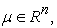scale parameter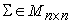and skewness parameter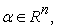denoted as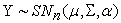, then the density function can be given by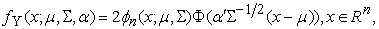(1)

where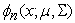is the n-dimensional normal density function with mean vector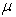and covariance matrix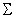, and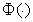is the standard normal distribution function.

In this paper, we apply the following properties of skew-normal distribution during parameter estimation of skew-normal mixed model (See 15 for more about the properties of skew-normal distribution).

Proposition 1 If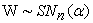, then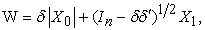(2)

where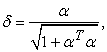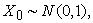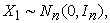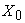and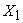are independent.

Lemma 1 If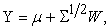where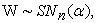thenand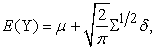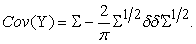Proposition 2 If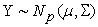,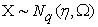, then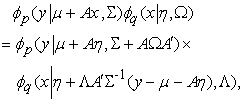where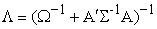.

### 3. Parameter Estimation

Due to the existence of redundant parameters in skew-normal mixed model, the maximum likelihood estimate gives no explicit solutions. Therefore, we apply the EM algorithm to give the maximum likelihood estimate of unknown parameters. As an iterative algorithm, the EM algorithm can be used to give the maximum likelihood estimate of parameters in probability models with implicit variables (See 16 for more about the EM algorithm). Then, the skew-normal mixed model is given as in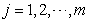(3)

where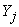is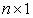random vector denoting the carbon intensity,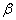and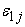respectively denote fixed and random effects.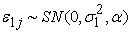and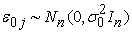are independent. According to the above-mentioned skew-normal distribution properties, we get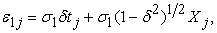where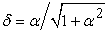,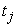is the absolute value of the standard normal distribution random variable,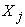is the random variable following standard normal distribution,andare independent. Therefore, (3) can be expressed as in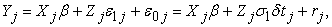where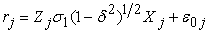. In the application of EM algorithm,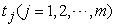are implicit variables. Let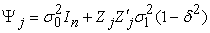, then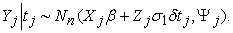(4)

Let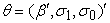, then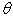and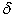are the parameters to be estimated in (3). Under Proposition 2 and (4), it follows that the joint distribution ofandcan be given by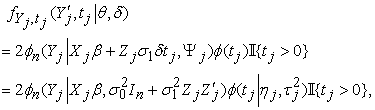where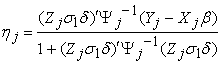and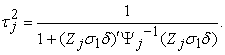And the log-likelihood function of (3) is given by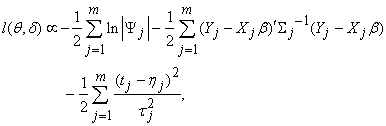where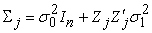.

The conditional distribution, first order and second order original moments ofwith regard tocan be obtained as in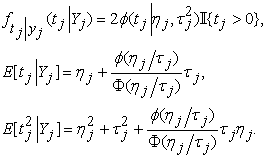(5)

Then, the following steps can be used to estimate the parameters of (3).

E-step: Given,and the parameters of the last iteration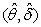, using (5) we can get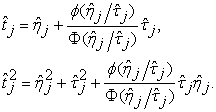M-step: Based on the estimated parameters of E-step, updateby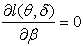, and get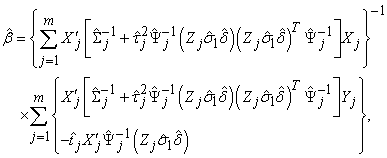where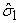,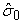and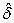are based on the previous iterations.

Given the original values of parameters to be estimated, which are often chosen to be the corresponding estimates under a normal assumption, we repeat E-step and M-step till convergence, and then obtain maximum likelihood estimate.

### 4. Empirical Analysis

4.1. Specification of Variables and Data

Our preliminary research show that carbon intensity is mainly influenced by factors including income elasticity of environmental quality demand, scale effects, technical effects and structure effects. Herein, we use the following independent variables.

GDP per capita, denoted by CG, represents the scale of economic activities.

Energy intensity, denoted by EI, refers to energy consumption per unit of GDP.

The proportion of secondary industry, denoted by IS, refers to the proportion of the second industry value added in GDP.

The dependence on foreign trade, denoted by FT, refers to the total value of commodity imports and exports with regard to GDP.

As for the dependent variable, carbon intensity denoted by CI refers to carbon emission per unit of GDP. And carbon emission is calculated using a carbon emission conversion method (See 17) and coefficients as in Table 1.

We use the longitudinal data of the above variables in 30 provinces of China from 2000 to 2014. Data are derived from China Statistical Yearbook and China Energy Statistics Yearbook.

4.2. Test of Skew-normal Distribution

Descriptive data analysis show that the mean, variance and skewness of carbon intensity is 0.223, 0.293 and 0.435 respectively, all in logarithm forms. Figure 1 gives the frequency distribution histogram. Further, results of Shapiro-Wilk test, Kolmogorov-Smirnov test and Cramer-von Mises test show that the p-values are 9.447e-05, 4.646e-05 and 3.642e-04 respectively, all significant at 5% significance level. These normality testing indicates that the carbon intensity data are not normally distributed.

View option
• Figure 1. Histogram of carbon intensity data

Based on (1) and the method of moment estimation, we find the carbon intensity data follows the skew-normal distribution SN(-0.32, 0.588, 1.932), the probability density curve of which is shown in Figure 1. In addition, results of the Chi-square goodness-of-fit test show that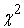= 10.502 <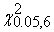= 12.592, so the null hypothesis of skew-normal distribution is not rejected at 5% significance level. Hence, the distribution of carbon intensity can be considered as skew-normal.

The above analysis verify the skew-normal distribution characteristics of carbon intensity in China. Meanwhile, since mixed models are most commonly used to fit longitudinal data, a skew-normal mixed model is then constructed for further analysis.

4.3. Parameter Estimation of Skew-normal Mixed Model

The above-mentioned maximum likelihood estimation method is adopted to construct the skew-normal mixed model as in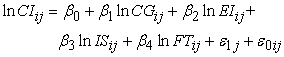(6)

where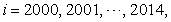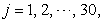random effects, error term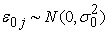.

Table 2 gives the estimation results of two mixed models under different assumptions that random effects follow skew-normal distribution and normal distribution respectively. Compared with the normal mixed model, the AIC and BIC values of the skew-normal mixed model dropped by 15.4 and 11.3, respectively. It is obvious that the skew-normal mixed model fits the longitudinal data better than the normal one.

We then further analyze the estimation results of the skew-normal mixed model, which can be summerized as in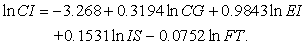(7)

GDP per capita, energy intensity and the proportion of secondary industry would have positive impacts on carbon intensity, while the dependence on foreign trade would negatively affect carbon intensity. With other conditions given, carbon intensity would rise by 0.32% as GDP per capita increases by 1%. An increase in energy intensity and secondary industry proportion by 1% would lead to an increase in carbon intensity by 0.98% and 0.15%, respectively. On the contrary, as dependence on foreign trade rises by 1%, carbon intensity would dropped by nearly 0.08%. It is found China's carbon intensity could be significantly cut down by reducing energy intensity and the proportion of second industry, as well as increasing the dependence on foreign trade. In addition, due to the positive impact of GDP per capita on carbon intensity, it is crucial that China transforms its current extensive mode of economic growth by which significant increases in carbon emissions are fundamentally determined.

These estimation results could be biased and unreliable, if we tried to fit our skewed data under the assumption of normal distribution. As shown in Table 2, the impacts of GDP per capita and energy intensity on carbon intensity would be overestimated, while the impacts of secondary industry proportion and foreign trade dependence would be underestimated.

### 5. Conclusions

This paper firstly discusses the parameter estimation method for skew-normal mixed model based on the EM algorithm. Then, for empirical analysis, the skew-normal distribution characteristics of longitudinal carbon intensity data are verified. A skew-normal mixed model is then constructed to study the main influencing factors of carbon intensity of China, the estimation results of which are compared with those based on normal mixed model. It is confirmed that the skew-normal mixed model fits the longitudinal carbon intensity data of China better than the normal model, and therefore have statistical excellent properties.

It is found that factors including GDP per capita, energy intensity, proportion of secondary industry, and dependence on foreign trade would have significant impacts on China’s carbon intensity. In particular, carbon intensity could be reduced by lowering energy intensity and proportion of secondary industry, as well as opening more to the world economy. Still, there exists harsh challenges as China accelerates the process of economic growth mode transformation, and fundamentally control carbon emission with a more intensive economic development. Accordingly, the following suggestions are put forward.

Firstly, our empirical analysis show that energy intensity is the most significant influencing factor of carbon intensity. And therefore, it would be the most direct and effective measure to reduce the carbon intensity by lowering energy intensity. To this end, the efficiency of energy utilization needs to be improved by strengthening R&D of new energy, energy conservation and emission reduction.

Secondly, since carbon intensity drops with the proportion of secondary industry, it makes sense that the secondary industry be reduced in a more carbon-emission-friendly way, and the industrial structure be optimized. The emission-intensive industries needs to be limited or upgraded. In fact, not only the secondary industry but also the economic growth mode of the whole country needs transformation urgently.

Thirdly, it would help to reduce carbon intensity by more opening-up in international trade. However, special attention needs to be paid to avoid the expansion of emission-intensive production and exportation. Therefore, the export of goods highly intensive in carbon emission and energy consumption should be under control, while the export of low-carbon goods could be supported.

### Acknowledgements

This research was supported by Zhejiang Provincial Philosophy and Social Science Planning Zhijiang Youth Project of China (16ZJQN017YB), National Natural Science Foundation of China (11401148), National Social Science Foundation of China (12CJY012), National Bureau of Statistics Key Project of China (2015LZ14), Outstanding Post-graduate Dissertation Cultivation Fund of Hangzhou Dianzi University (yxlw2016014), and Outstanding Post-graduate Dissertation Cultivation Foundation of Zhejiang Provincial University Humanities and Social Science Key Research Base (201627).

### References

  Y.G. Zhang, Economic development pattern change impact on China’s carbon intensity, Economic Research Journal, 2010, (4): 120-133. In article  Y.Z. Xu, K.N. Xu, Y.S. Hu, Driving factors and decoupling effect of carbon emissions: evidence from China's manufacturing sector, Statistical Research, 2011 (7), 55-61. In article  J.W. Xu, Study on the factors of China's carbon dioxide emissions based on LMDI, Doctor Degree Dissertation, China University of Mining and Technology (Beijing), 2013. In article  D. Li, C.X. Wang, Panel data analysis on the relationship between China's economic growth and air pollution, Finance and Economics , 2007, (2): 43-50. In article  Y.H. Yu, X.Y. Zheng, L. Zhang, Carbon dioxide emission and economic development: a panel data anaalysis, Economic Theory and Business Management, 2011, (3): 72-81. In article  Y.F. Qi, Convergence and divergence analysis of China’s regional economic growth and carbon emissions’ district differences, Master Degree Dissertation, Xiangtan University, 2012. In article  G. Verbeke, E. Lesaffre, The effect of misspecifying the random effects distribution in linear mixed models for longitudinal data, Computational Statistics and Data Analysis, 1997, 23(4): 541-556. In article View Article  W. Ghidey, E. Lesaffre, P. Eilers, Smooth random effects distribution in a linear mixed model, Biometrics, 2004, 60(4): 945-953. In article View Article  PubMed  T.I. Lin, J.C. Lee, Estimation and prediction in linear mixed models with skew normal random effects for longitudinal data, Statistics in Medicine, 2008, 27(9): 1490-1507. In article View Article  PubMed  D. Zhang, D. Marie, Linear mixed models with flexible distributions of random effects for longitudinal data, Biometrics, 2001, 57: 795-802. In article View Article  PubMed  V.H. Lachos, P. Ghosh, R.B. Arellano-Valle, Likelihood based inference for skew-normal independent linear mixed models, Statistica Sinica, 2010, 20(1): 303-322. In article View Article  R.D. Ye, T. Wang, Inferences in linear mixed models with skew-normal random effects, Acta Mathematica Sinica (English Series), 2015, 31(4): 576-594. In article View Article  S.W. Meng, H. Z. Xiao, Credibility premium based on skew-normal random effect model, Statistical Research, 2015, (1): 73-78. In article View Article  A. Azzalini, A class of distributions which includes the normal ones, Scandinavian Journal of Statistics, 1985, 12(2): 171-178. In article View Article  R.B. Arellano-Valle, H. Bolfarine, V.H. Lachos, Skew-normal linear mixed models, Journal of Data Science, 2005, 3(4): 415-438. In article View Article  A.P. Dempster, N.M. Laird, D.B. Rubin, Maximum likelihood from incomplete data via the EM algorithm, Journal of the Royal Statistical Society (Series B), 1977, 39(1): 1-38. In article View Article  X.Y. Li, X.M. Wu, J.Q. Ma, The measure of China’s industry carbon emissions and the structural decomposition analysis of influencing factors, Statistical Research, 2014, (1): 56-62. In article View Article

Published with license by Science and Education Publishing, Copyright © 2018 Ye Ren-dao, Xu Li-jun, Luo Kun and Zhang YongThis work is licensed under a Creative Commons Attribution 4.0 International License. To view a copy of this license, visit http://creativecommons.org/licenses/by/4.0/